Variables

This category contains 16 nodes.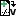Create File Name

Create a new file name as flow variable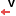Extract Variables (Data)

Extracts Variables from a data connection.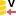Extract Variables (Database)

Extracts Variables from a Database connection.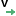Inject Variables (Data)

Merges Variables from one connection into the data connection. The data is simply handed through.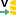Inject Variables (Database)

Merges Variables from one connection into the database connection. The database is simply handed through.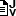Java Edit Variable

Edit or add flow variables using java code.Java Edit Variable (simple)

Edit a flow variable using java code.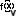Math Formula (Variable)

Evaluates mathematical formula, appending result as a new variable or replacing an input variable.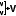Merge Variables

Merges flow variables into one stream.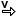Rule Engine Variable

Applies user-defined business rules to the flow variables